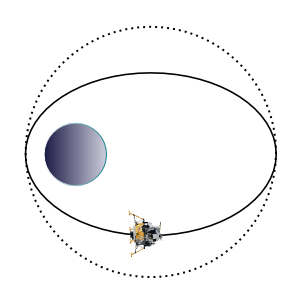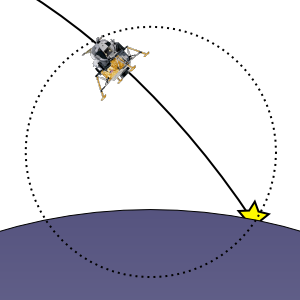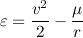## Monday, October 29, 2007

### Auto-zooming view

This week I started by trying to improve my powered-landing cutoff elevation by using the correct equation for gravitational acceleration as a function of altitude:This is a differential equation; the acceleration due to gravity is a function of the inverse squared distance above the planet. Mu is a constant based on the size and density of the planet. I was attempting to come up with an equation for y given t. Unfortunately I didn't get anywhere with it. I'm not sure if it has a closed-form solution or not.

After that I turned to a couple of other parts of my lander program that needed improvement. The first was computation of the impact site. Because I am currently assuming all the gravity comes from the one planet, the spacecraft trajectory is a conic section (ellipse, parabola, or hyperbola). I can thus do an analytical intersection between the trajectory curve and the circle of the planet's surface.

My old code was an ellipse/circle intersection. It thus couldn't handle parabolic or hyperbolic trajectories. It also suffered from numerical accuracy problems right around takeoff and landing, when the trajectory is just barely larger than the circle and has an eccentricity near one.

I switched to the polar representation for a conic:p is the semi-latus rectum, a measure of the size of the conic. e is the eccentricity, a measure of the shape of the conic.

This handles hyperbolic and parabolic trajectories just fine. Its problem is that it falls down when the trajectory becomes degenerate. When you take off vertically, the trajectory follows a straight line. In this case, p is 0, so the equation returns 0 for the radius no matter what angle theta you put in. So, I need to switch to a different algorithm in those cases. I still need to do that.

The third thing I worked on was my view-framing algorithm. I try to zoom the camera in and out so that you can see what's important. When you're in orbit, I frame the entire orbit:Once you are on a collision trajectory, I want to frame the unflown portion of the trajectory:Once you've landed, I want to have a close-up view of the spacecraft:There are still some cases that aren't covered. For instance, what should I frame when the trajectory is hyperbolic? In this case it's infinite. I currently cap the amount that I will zoom out, but if the spacecraft exceeds the limit I zoom out further.

It turns out that the specific mechanical energy is a good measure to use for identifying the different situations. It's the sum of the kinetic energy and the potential energy, divided by the spacecraft's mass:The kinetic energy is the first term; you probably recognize it (minus the mass) from physics textbooks. The potential energy is the second term; it's set up such that it is zero when the spacecraft is infinitely far away, and gets more negative as the spacecraft approaches the planet.

The specific mechanical energy stays constant as the spacecraft moves around a given trajectory. For non-circular trajectories, potential energy is traded with kinetic energy as the spacecraft orbits.

When the specific mechanical energy is positive, the spacecraft is on a hyperbolic trajectory. When it is zero, the trajectory is parabolic. Negative means an elliptic orbit. The size of the orbit is related to the energy. I use the energy in a low circular orbit as the cutoff point for blending between a landing camera and an orbit camera. Since the energy changes smoothly as the player fires the rockets, it is a good parameter for interpolating between camera modes.Fundamentals of Astrodynamics, by Bate, Mueller, and White, is the book I am dog-earing as I work through this stuff. It's an unbeatable value, as is typical of Dover books. If you are at all interested in astrodynamics it's a must-have.